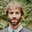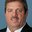Question-and-Answer Resource for the Building Energy Modeling Community
Get started with the Help page

# How to calculate HVAC Electric demand power correctly?Hello, I'm studying EnergyPlus and made two-story house model by sketchup. I'm using EnergyPlus to calculate temperature, heating energy and electric power consumption.

I put AC(25℃) 5hours and put internal gains (light, people, electric equipment) and simulated. As a result, AC worked correctly, but "HVAC electric demand power(W)" was strange. I thought electric demand power get big when AC is working, but it didn't change at all. Then I thought ventilation making HVAC electric demand power too high, so I deleted ventilation once and simulated again, but it also didn't change.

Could somebody tell me which option making an effect to HVAC Electric demand power? And tell me how to decrease electric power consumption of HVACs? (In my present simulation result, HVAC electric demand power is about 1119～1120W/h)

edit retag close merge delete

Sort by » oldest newest most votedEnergyPlus actually aggregates energy use of building components. The output variable for HVAC Electric Demand is calculated from the energy consumption of all HVAC equipment on the Electricity:HVAC meter.

From the rdd file:

Output:Variable,*,Facility Total HVAC Electric Demand Power,hourly; !- HVAC Average [W]


This report variable is the average power over the time step being reported, in this case 1 hour. To find which HVAC equipment is included in the Electricity:HVAC meter, look in the mtd file and search for Electricity:HVAC. For the example file FurnaceWithDXSystem you would see this:

For Meter=Electricity:HVAC [J], ResourceType=Electricity, Group=HVAC, contents are:
SUPPLY FAN 1:Fan Electric Energy
FURNACE HEATING COIL 1:Heating Coil Electric Energy
FURNACE ACDXCOIL 1:Cooling Coil Electric Energy


These components are on the Electricity:HVAC electrical energy meter. To get power demand from this data you would divide energy (Joules) by the number of seconds in the reported time step. For hourly reports that would be 3600 seconds. However, reporting power demand is less accurate when averaging electricity data over long time periods because power demand varies by the second. In EnergyPlus you can select shorter time steps and report at the TimeStep frequency. If you select the number of time steps in hour to be 4 you would be simulating over a 15-min time period.

Timestep,
4;              !- Number of Timesteps per Hour


To calculate power from the electrical energy meters you would divide the electrical energy use in Joules by the number of seconds in the time step:

Power (W) = Electrical Energy (Joules per Timestep) / (3600 seconds per hour / Timesteps per hour)

or

Power (W) = Electrical Energy (J) * Number of Timestep per hour / 3600 (seconds per hour) = J/s


If your HVAC system is on the entire time step then you will see the total power output of the HVAC system. If the HVAC system operates only a portion of the time step then the electrical energy use should be an average over the time step and the magnitude of power should be lower than the maximum.

more